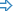# Working With Excel Files via COMSometimes, you may not be able to use the `Excel` object to work with Excel files. For example, you cannot use the object to work with ranges of cells, change cell formats, or work with cells containing formula expressions. In this case, you can work with Excel files by using the Excel COM server — `Excel.Application`. To get it in your tests, use the `Sys.OleObject` property or, if you use JavaScript, the `getActiveXObject` method.

For more information about the Excel OLE object model, see the Excel Object Model Overview article in the MSDN library.

The following code illustrates how you can read data from Excel cells by using the Excel OLE object and post this data to the TestComplete log:

JavaScript

{
let Excel = getActiveXObject("Excel.Application");
Excel.Workbooks.Open("C:\\MyFile.xlsx");

let RowCount = Excel.ActiveSheet.UsedRange.Rows.Count;
let ColumnCount = Excel.ActiveSheet.UsedRange.Columns.Count;

for (let i = 1; i <= RowCount; i++)
{
let s = "";
for (let j = 1; j <= ColumnCount; j++)
s += (VarToString(Excel.Cells.Item(i, j)) + "\r\n");
Log.Message("Row: " + i, s);
}

Excel.Quit();
}

JScript

{
var Excel = Sys.OleObject("Excel.Application");
Excel.Workbooks.Open("C:\\MyFile.xlsx");

var RowCount = Excel.ActiveSheet.UsedRange.Rows.Count;
var ColumnCount = Excel.ActiveSheet.UsedRange.Columns.Count;

for (var i = 1; i <= RowCount; i++)
{
var s = "";
for (var j = 1; j <= ColumnCount; j++)
s += (VarToString(Excel.Cells(i, j)) + "\r\n");
Log.Message("Row: " + i, s);
}

Excel.Quit();
}

Python

``````def ReadDataFromExcel():
Excel = Sys.OleObject["Excel.Application"]
Excel.Workbooks.Open("C:\\MyFile.xlsx")

RowCount = Excel.ActiveSheet.UsedRange.Rows.Count
ColumnCount = Excel.ActiveSheet.UsedRange.Columns.Count

for i in range(1, RowCount + 1):
s = "";
for j in range(1, ColumnCount + 1):
s = s + VarToString(Excel.Cells.Item[i, j]) + '\r\n'
Log.Message("Row: " + VarToString(i), s);

Excel.Quit();``````

VBScript

Dim Excel, RowCount, ColumnCount, i, j, s

Set Excel = Sys.OleObject("Excel.Application")
Excel.Workbooks.Open("C:\MyFile.xlsx")

RowCount = Excel.ActiveSheet.UsedRange.Rows.Count
ColumnCount = Excel.ActiveSheet.UsedRange.Columns.Count

For i = 1 To RowCount
s = ""
For j = 1 To ColumnCount
s = s & VarToString(Excel.Cells(i, j)) & vbNewLine
Next
Log.Message "Row: " & i, s
Next

Excel.Quit
End Sub

DelphiScript

var Excel, RowCount, ColumnCount, i, j, s;
begin
Excel := Sys.OleObject('Excel.Application');
Excel.Workbooks.Open('C:\MyFile.xlsx');

RowCount := Excel.ActiveSheet.UsedRange.Rows.Count;
ColumnCount := Excel.ActiveSheet.UsedRange.Columns.Count;

for i := 1 to RowCount do
begin
s := '';
for j := 1 to ColumnCount do
s := s + VarToString(Excel.Cells(i, j)) + #13#10;
Log.Message('Row: ' + VarToString(i), s);
end;

Excel.Quit;
end;

C++Script, C#Script

{
var Excel = Sys["OleObject"]("Excel.Application");
Excel["Workbooks"]["Open"]("C:\\MyFile.xlsx");

var RowCount = Excel["ActiveSheet"]["UsedRange"]["Rows"]["Count"];
var ColumnCount = Excel["ActiveSheet"]["UsedRange"]["Columns"]["Count"];

for (var i = 1; i <= RowCount; i++)
{
var s = "";
for (var j = 1; j <= ColumnCount; j++)
s += (VarToString(Excel["Cells"](i, j)) + "\r\n");
Log["Message"]("Row: " + i, s);
}

Excel["Quit"]();
}

### Samples

TestComplete includes a sample that demonstrates how to work with data stored in Excel files from scripts via the `Excel.Application` COM object:<TestComplete Samples>\Common\MSOffice\

 Note: If you do not have the sample, download the TestComplete Samples installation package from the support.smartbear.com/testcomplete/downloads/samples page of our website and run it.

To find more samples that demonstrate how to work with Excel data via its COM server in TestComplete, follow the link below:http://www.sqaforums.com/showflat.php?Cat=0&Number=345470&an=0&page=0&gonew=1#UNREAD